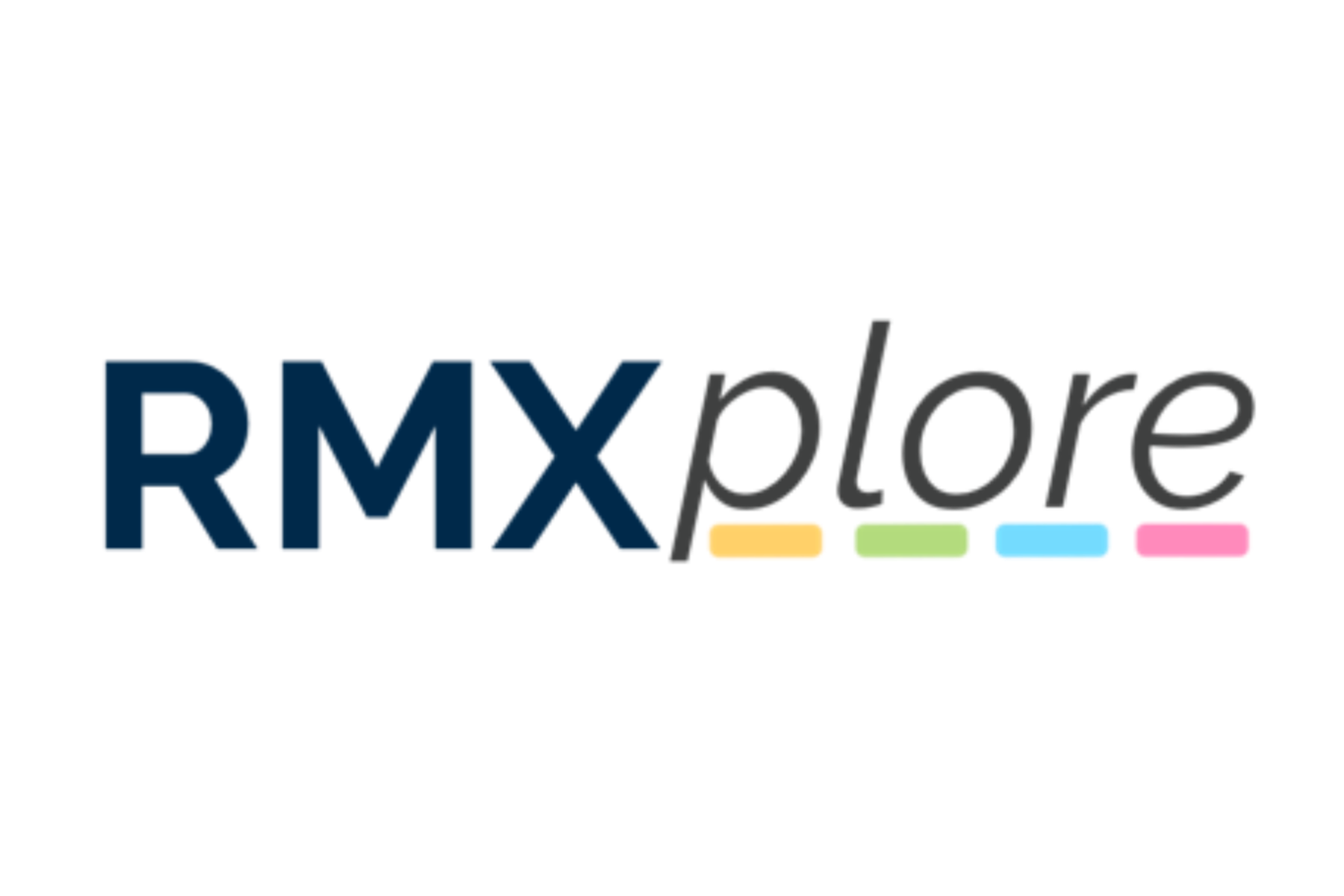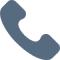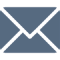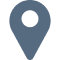# Operations, Measurement, and Thinking (OMT) IV

Please note that RMXplore's Math Bytes Courses are not
currently open for registration
.

### Operations, Measurement, and Thinking (OMT) IV

Coming Soon!
Course Pricing:
• Online : \$260
• Houston Center : \$320
• Cupertino Center : \$400
Course Logistics:
Duration : to be updated
Number of Classes: 5 weeks course
Occurs : to be updated

### Math Byte Course Topics:

Course Topics are organized as six modules with five granular topics covered under each module. Click through below to see all modules/topics for this course.

#### 01 - Additions and Subtraction• Comparing integers
• Multiplication with fraction
• Divisions with fractions
• Decimals into fractions
• Operations with Decimals

#### 02 - Multiplication and Division• Comparing integers
• Multiplication with fraction
• Divisions with fractions
• Decimals into fractions
• Operations with Decimals

#### 03 - Fractions• Build solids out of unit cubes
• Side length of a cube given its volume/surface area
• Prisms: base area and volume
• Depth of water in a tank
• Changes to water levels

#### 04 - Measurement• Properties of parallelogram, rhombus and trapezium
• Angles in Polygons
• Construction of 2-D shapes
• 2-D representation (nets) of common 3-D objects
• Identifying the solid that can be formed from a given net

#### 05 - Elementary Geometry• Introduction to pie charts
• Drawing pie charts
• Interpreting pie charts
• Categorizing pie charts
• Other types of data visualization

#### 06 - Word Problems• Applications of Percentages
• Ratios and Proportions
• Work and Rate
• Missing angles, area, and volume
• Applying data analysis techniques (Pie chart)